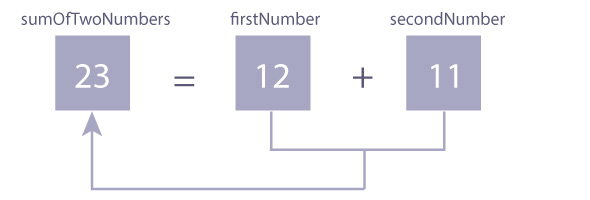Friday, 19 May 2017 04:32

Written by
Rate this item

## Program to Add Two Integers

``````#include <stdio.h>
int main()
{
int firstNumber, secondNumber, sumOfTwoNumbers;

printf("Enter two integers: ");

// Two integers entered by user is stored using scanf() function
scanf("%d %d", &firstNumber, &secondNumber);

// sum of two numbers in stored in variable sumOfTwoNumbers
sumOfTwoNumbers = firstNumber + secondNumber;

// Displays sum
printf("%d + %d = %d", firstNumber, secondNumber, sumOfTwoNumbers);

return 0;
}``````

Output

```Enter two integers: 12
11
12 + 11 = 23
```

In this program, user is asked to enter two integers. Two integers entered by the user is stored in variables firstNumber and secondNumber respectively. This is done using `scanf()`function.

Then, variables firstNumber and secondNumber are added using + operator and the result is stored in sumOfTwoNumbers.Finally, the sumofTwoNumbers is displayed on the screen using `printf()` function.